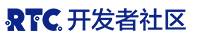分解过程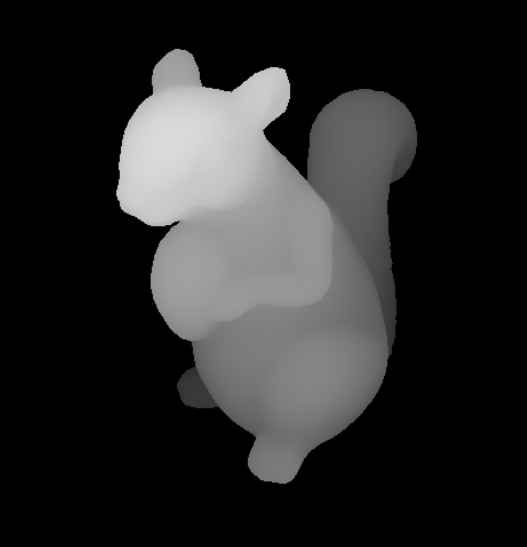如何编码这个工具

import os, sys
import numpy as np
import pygame as pg
import cv2

depth_map = cv2.cvtColor(depth_map,cv2.COLOR_RGB2GRAY)
img = cv2.resize(img, depth_map.shape[:2])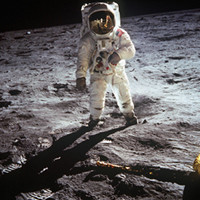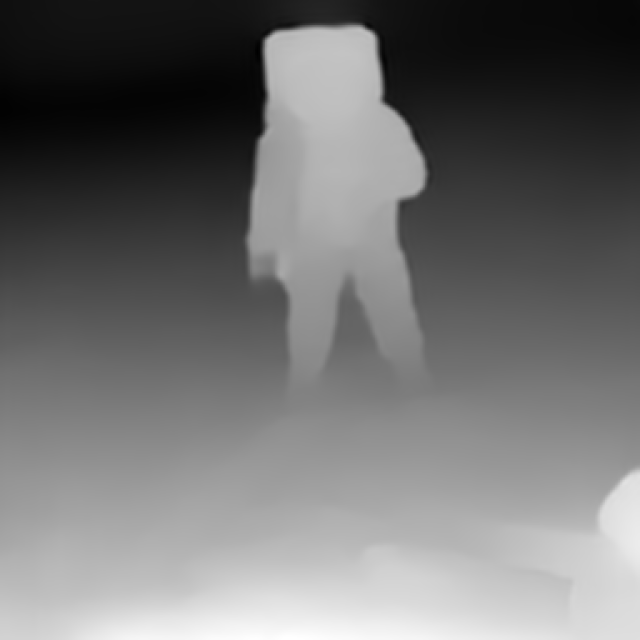b, g, r = cv2.split(cv_image)
rgba = [r, g, b, mask]
cv_image = cv2.merge(rgba,4)

return cv_image

def get_face_rect(img):
gray_img = cv2.cvtColor(img, cv2.COLOR_BGR2GRAY)
if len(face_rects) == 0:
return ()
return face_rects

scale = 1
off_set = 20
width, height = layers.get_width(), layers.get_height()        win = pg.display.set_mode((int((width - off_set)*scale), int((height - off_set)*scale)))
pg.display.set_caption('Parallax_image')
scaled_layers = []
for layer in layers:
scaled_layers.append(pg.transform.scale(layer, (int(width*scale), int(height*scale))))
cap = cv2.VideoCapture(0, cv2.CAP_DSHOW)

x_transform = True     # allow shift in x-axis
y_transform = False    # allow shift in y-axis
sens = 50              # the amount of scale down of shift value
show_cam = False       # show your face cam
shift_x = 0
shift_y = 0
run = True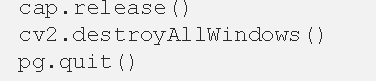https://miro.medium.com/max/863/1H6L0UFuTpWzD_IB0EFqSvg.gif

https://miro.medium.com/max/863/1
24XN55FuLp0IKIa3PQefPw.gif